# Object detection - class review

Last updated on：a year ago

Localization and detection can be used image classification, classification with localization and multiple object detection.

# Output of object detection network

$$y = [P_c, b_x, b_y, b_h, b_w, c_1, c_2, c_3 ]^T$$
If $$P_c: \text{is there any} c_y \text{object}$$

$$L (\hat{y}, y) = (\hat{y}_1 - y_1)^2 + (\hat{y}_2 - y_2)^2 + … + (\hat{y}_8 - y_8)^2, if y_1 = 1(\hat{y}_1 - y_1)^2 \text{if} y_1 = 0$$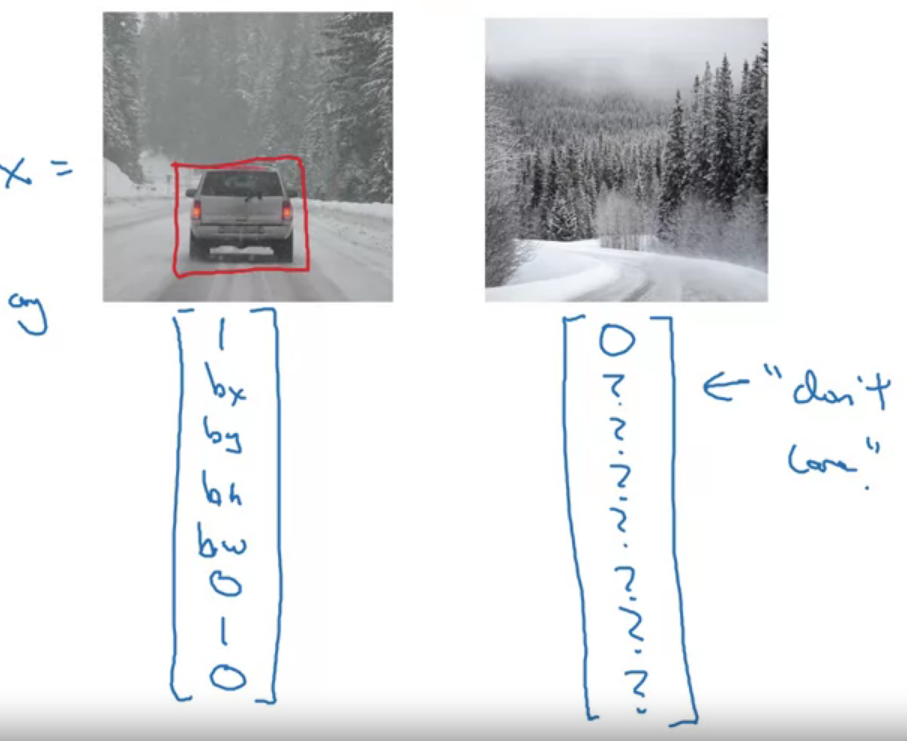# Landmark detection

Select the number of landmarks.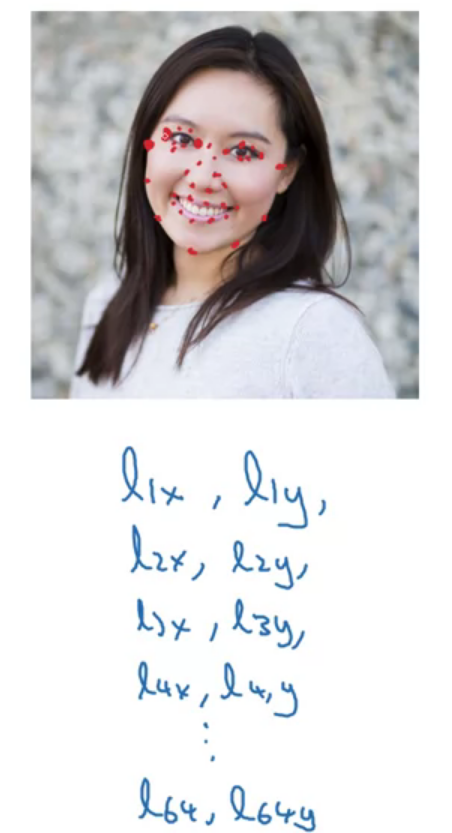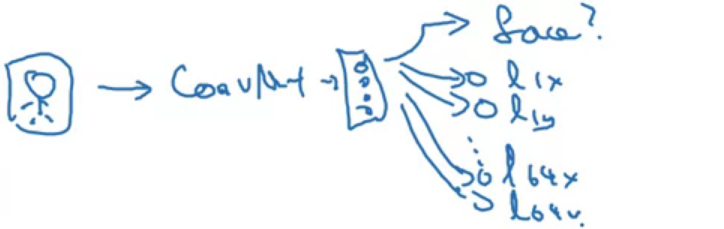129 output units = $64 \times 2 + 1$

Analyse the pose of the person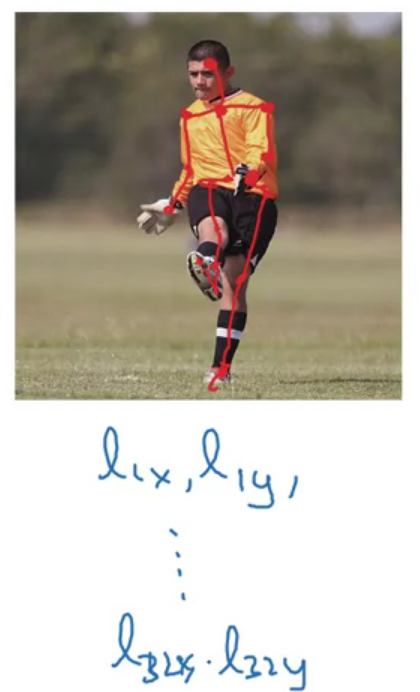# Object detection

Sliding windows detection

You then repeat it, but now use a larger window.

You can reduce the number of windows you need to pass through the confinite by using a more coarse stride, a bigger stride, a larger step size. The sliding detector can be implemented convolutionally, or much more efficient.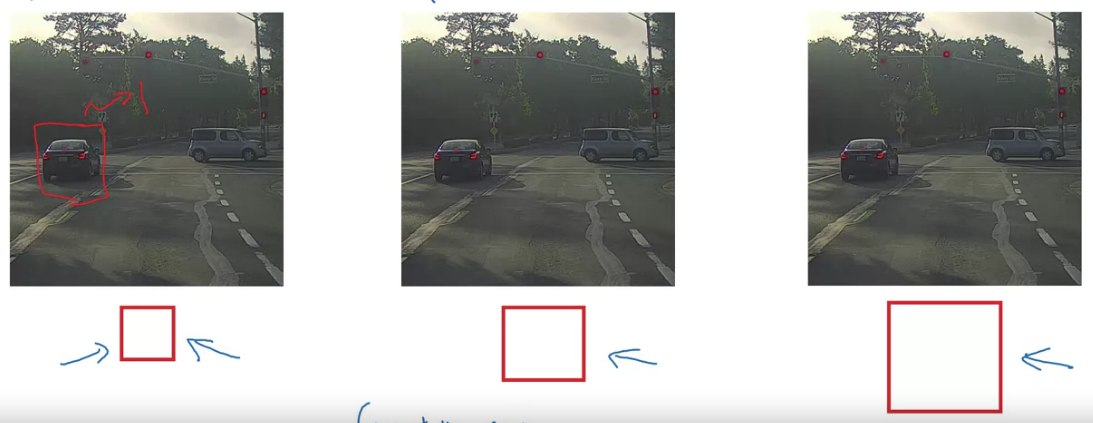Turning FC layer into convolutional layers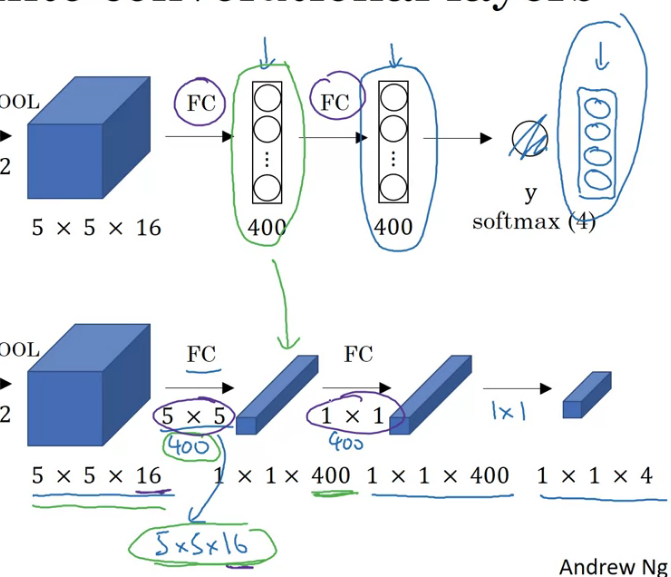Convolutional implementation of sliding windows

Problem: the position of the bounding boxes are not too accurate.

Bounding boxes predictions
output accurate bounding boxes.

# Reference

 Deeplearning.ai, Convolutional Neural Networks